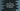# 2 different C programs to find the volume and surface area of a cylinder## C program to find the volume and surface area of a cylinder:

In this C program, we will learn how to find the volume and surface area of a cylinder. Let’s learn how to find the surface area and volume of a cylinder before we start writing the program.

### How to find the volume and surface area of a cylinder:

We need the height and radius of a cylinder to find the volume and surface area of a cylinder. For example, if h is the height and r is the radius of a cylinder,

The surface area will be: `2πr² + 2πrh` The volume will be: `πr²h`

Where π is the mathematical constant PI.

The program will take the radius and height of the cylinder as the inputs from the user and it will calculate and print the surface area and volume of the cylinder.

### Example 1: C program to find the volume and surface area of a cylinder:

The below program takes the height and radius of the cylinder as inputs from the user and calculates the volume and surface area:

``````#include <stdio.h>
#include <math.h>

int main()
{

printf("Enter the height of the cylinder: ");
scanf("%f", &height);

printf("Enter the radius of the cylinder: ");

surfaceArea = 2 * M_PI * radius * radius + 2 * M_PI * radius * height;

printf("Volume: %.2f\n", volume);
printf("Surface Area: %.2f\n", surfaceArea);
return 0;
}``````

Here,

• Floating point variables height, radius, volume and surfaceArea are initialized to hold the height, radius, volume and surface area of the cylinder.
• It reads the height and radius of the cylinder as inputs from the user.
• It calculates the volume and surface area of the cylinder by using the same formulae we have discussed before. We are using math.h to use the PI value. M_PI is a predefined constant and this holds the value of PI.
• The last two lines are printing the calculated volume and surface area.

If you run this program, it will print output as below:

``````Enter the height of the cylinder: 5
Enter the radius of the cylinder: 4
Volume: 251.33
Surface Area: 226.19

Enter the height of the cylinder: 6
Enter the radius of the cylinder: 2
Volume: 75.40
Surface Area: 100.53``````

### Example 2: C program to find the volume and surface area of a cylinder by using a separate method:

We can use a separate method to find the volume and surface area of a cylinder. This method can be called from a different class as well. Let’s change the above program to use two separate methods to calculate the volume and surface area of a cylinder:

``````#include <stdio.h>
#include <math.h>

{
}

{
}

int main()
{

printf("Enter the height of the cylinder: ");
scanf("%f", &height);

printf("Enter the radius of the cylinder: ");